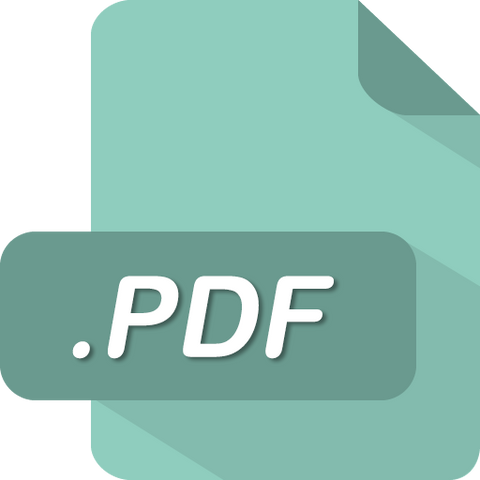# BUSI 530 BUSI530 Module 7 Homework 7 (Liberty University)

soffix

• \$9.99

BUSI530 Module 7 Homework 7 (Liberty University)

Question 1

Shares in Raven Products are selling for \$60 per share. There are 1 million shares outstanding. What will be the share price in each of the following situations? Ignore taxes. (Do not round intermediate calculations.)

Question 2

The stock of Payout Corp. will go ex­dividend tomorrow. The dividend will be \$0.55 per share, and there are 16,000 shares of stock outstanding. The market­value balance sheet for Payout is shown on the following table. Ignore taxes.

Question 3

Good Values, Inc., is all­equity­financed. The total market value of the firm currently is \$240,000, and there are 3,000 shares outstanding. Good Values plans to repurchase \$24,000 worth of stock. Ignore taxes.

Question 4

Investors require an after­tax rate of return of 13% on their stock investments. Assume that the tax rate on dividends is 30% while capital gains escape taxation. A firm will pay a \$3 per share dividend 1 year from now, after which it is expected to sell at a price of \$22.

Question 5

The expected pretax return on three stocks is divided between dividends and capital gains in the following way:

Question 6

Find the sustainable and internal growth rates for a firm with the following ratios: asset turnover = 1.60; profit margin = 5%; payout ratio = 40%; equity/assets = 0.40. (Do not round intermediate calculations. Round your answers to 2 decimal places.)

Question 7

Here are the abbreviated financial statements for Planners Peanuts:

Question 8

Here are the abbreviated financial statements for Planners Peanuts:

Question 9

ABC company financial manager believes that sales in 2012 could rise by as much as 20% or by as little as 5%.

Question 10

The following tables contain financial statements for Dynastatics Corporation. Although the company has not been growing, it now plans to expand and will increase net fixed assets (that is, assets net of depreciation) by \$3,800,000 per year for the next 5 years and forecasts that the ratio of revenues to total assets will remain at 1.50. Annual depreciation is 10% of net fixed assets at the end of 2012. Fixed costs are expected to remain at \$69,000 and variable costs at 80% of revenue. The company’s policy is to pay out two­thirds of net income as dividends and to maintain a book debt ratio of 25% of total capital.

Question 11

Plank’s Plants had net income of \$6,000 on sales of \$70,000 last year. The firm paid a dividend of \$600. Total assets were \$300,000, of which \$150,000 was financed by debt.

Sale

Unavailable

Sold Out